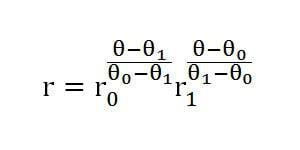# Equiangularity of a Polar Equation

• daisukekazama

## Homework Statement

Show that the equation below connects the point $$(r_{0}, \theta_{0})$$ to the point $$(r_{1}, \theta_{1})$$, $$\theta_{0}\neq\theta_{1}$$, along a curve that everywhere forms the same angle with the rays $$\theta=constant$$.

And here's the equation. I can't get the Latex to work... no clue why.N/A.

## The Attempt at a Solution

This is part of a larger problem on stereographic projection - I've figured out that this equation, if projected onto a sphere, traces out a loxodrome. I also know that I essentially have to prove that that mess of an equation is a equiangular spiral (logarithmic spiral).

The problem is, no matter how I look at it, I need to calculate the derivative $$dr/d\theta$$, which - from where I'm looking - looks like one hell of a messy and convoluted derivative, which I'd rather not do.

Is there an easier, cleaner, more elegant way of solving this problem than brute-forcing the derivative? If not, how do I do the derivative?

Then you are left simply in the form $$r= C r_0^{t\theta} r_1^{-t\theta}$$ and apply the product rule.